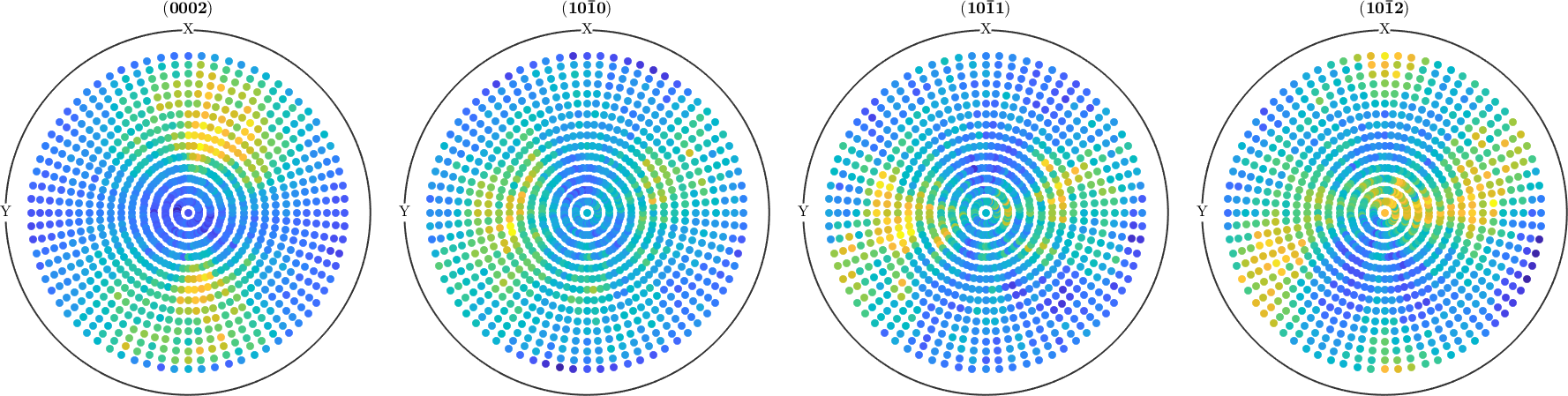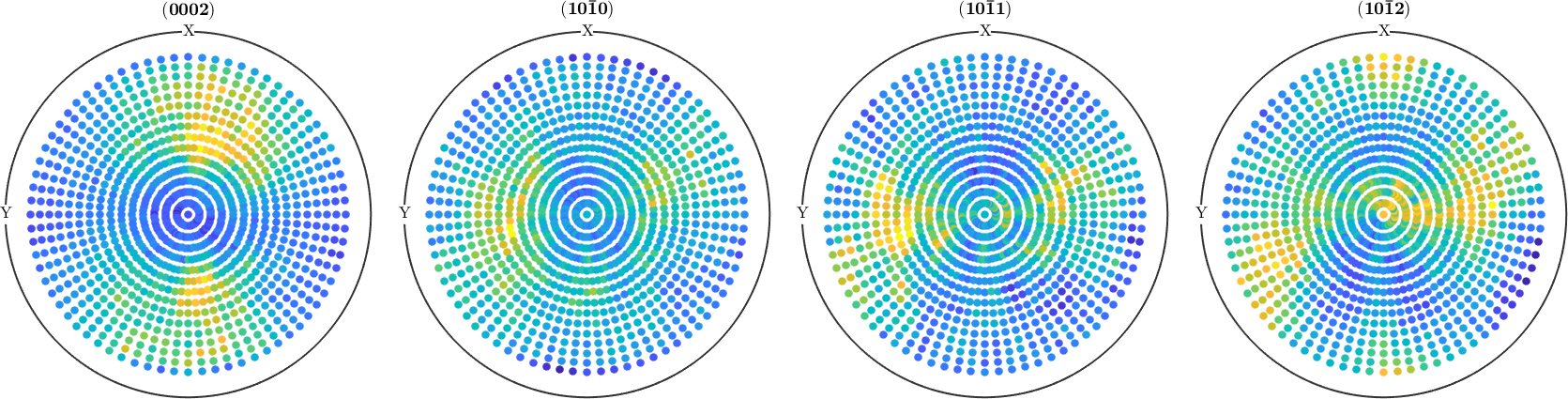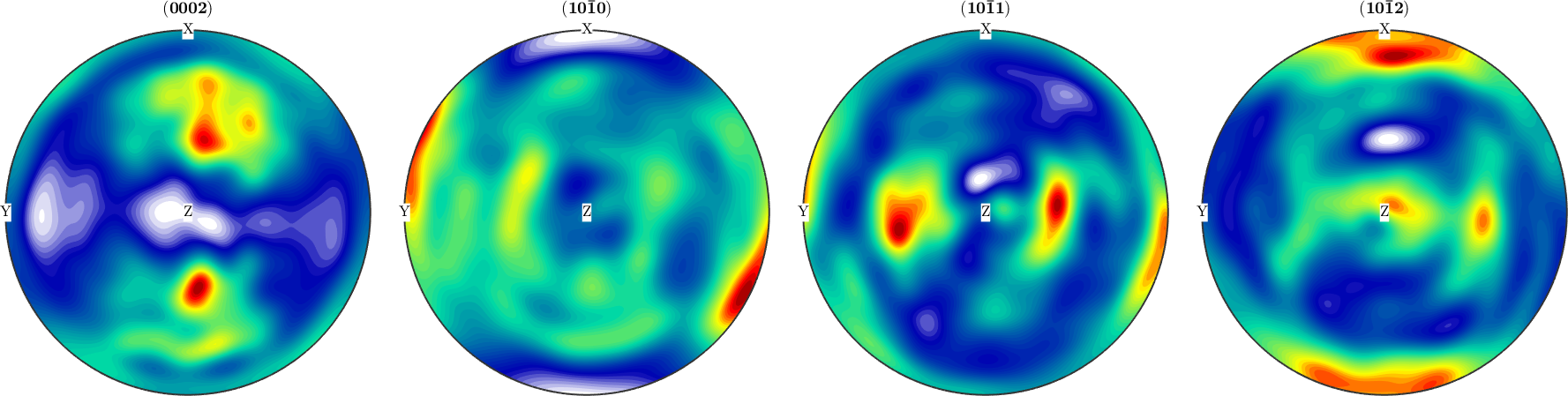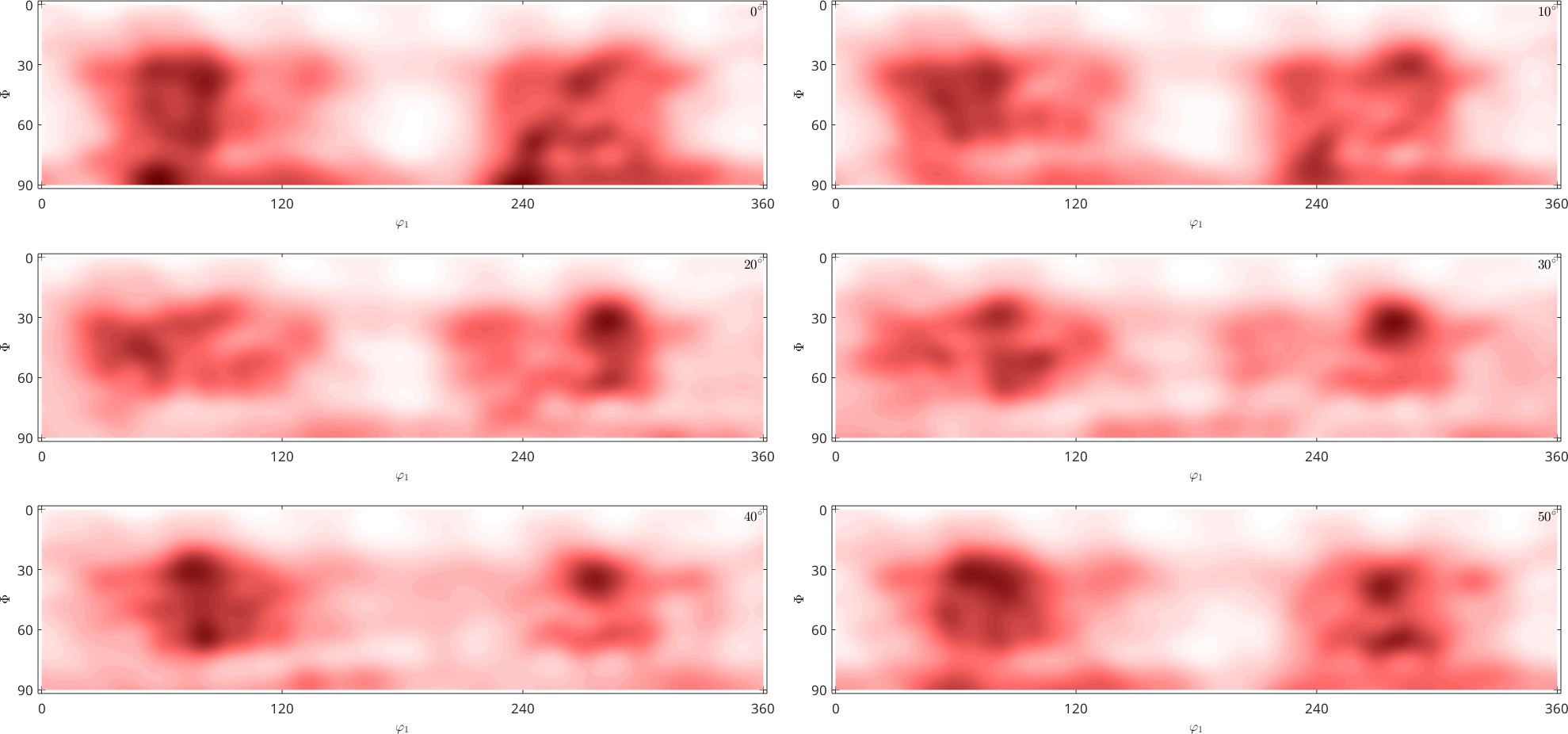Pole Figure Tutorial edit page

This tutorial explains the basic concepts for ananyzing x-ray, synchrotron and neutron diffraction pole figure data.

## Import pole figure diffraction data

Click on Import pole figure data to start the import wizard which is a GUI leading you through the import of pole figure data. After finishing the wizard you will end up with a script similar to the following one.

Specify File Names

Specify Miller Indices

Import the Data

After running the script the variable pf is created which contains all information about the pole figure data. You may plot the data using the command plotBy default pole figures are plotted as intensity-colored dots for every data point. There are many options to specify the way pole figures are plotted in MTEX. Have a look at the plotting section for more information.

After import make sure that the Miller indices are correctly assigned to the pole figures and that the alignment of the specimen coordinate system, i.e., X, Y, Z is correct. In case of outliers or misaligned data, you may want to correct your raw data. Have a look at the correction section for further information. MTEX offers several methods correcting pole figure data, e.g.

• rotating pole figures
• scaling pole figures
• finding outliers
• removing specific measurements
• superposing pole figures

As an example we set all negative intensities to zero## ODF Estimation

Once your data is in good shape, i.e. defocusing correction has been done and few outliers are left you can reconstruct an ODF out of this data. This is done by the command calcODF.

Note that reconstructing an ODF from pole figure data is a severely ill- posed problem, i.e., it does not provide a unique solution. A more through discussion on the ambiguity of ODF reconstruction from pole figure data can be found here. As a rule of thumb: the more pole figures you have and the more consistent your pole figure data the better your reconstructed ODF will be.

To check how well your reconstructed ODF fits the measured pole figure data useCompare the recalculated pole figures with the measured data. A quantitative measure for the fitting is the so called RP value. They can be computed for each imported pole figure with

In the case of a bad fit, you may want to tweak the reconstruction algorithm. See here for more information.

## Visualize the ODF

Finally one can plot the resulting ODF